# 手把手教你找回加密程序的密码

## 0×01 前言## 0×02 猜想

1.密码的明文；

2.密码的散列值；

3.使用密码和某一特征值生成对比密钥；

4.使用密码和用户待加密的原数据生成对比密钥；

5.………………

## 0×03 信息收集

### 第一步：样本设置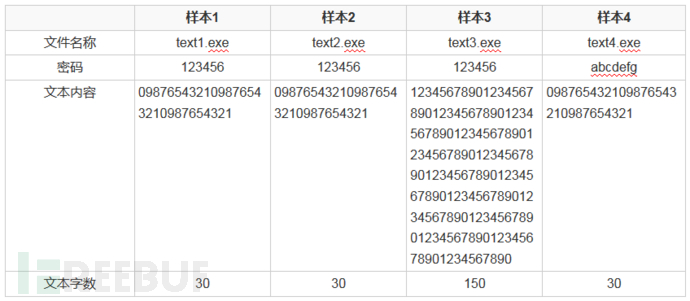### 第二步：文件静态分析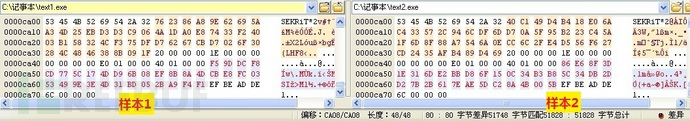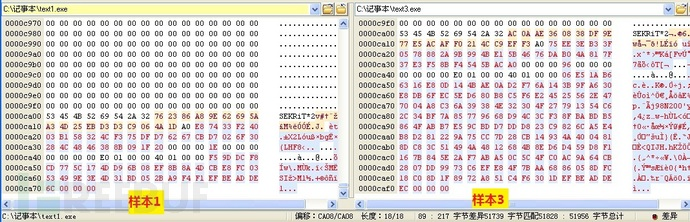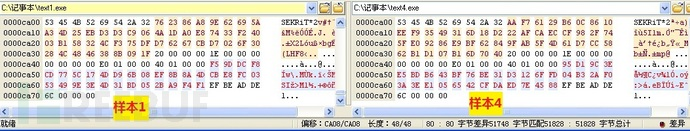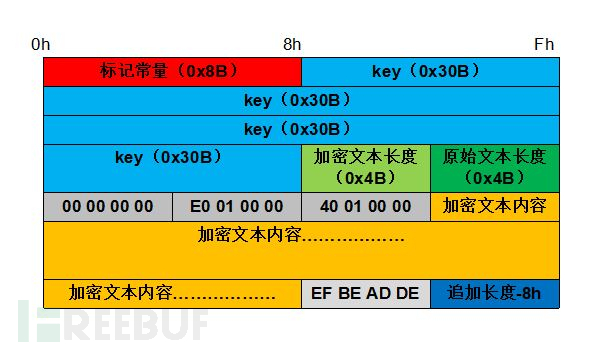### 第三步：IDA静态分析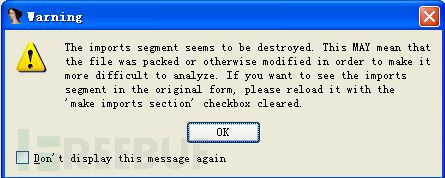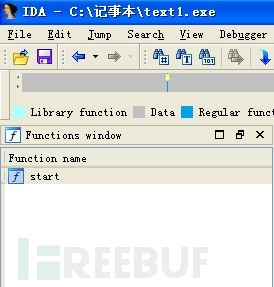### 第四步：脱壳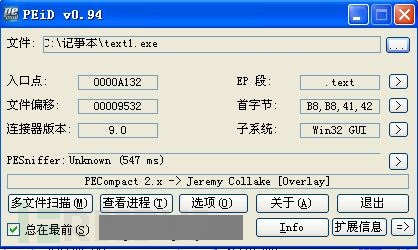PEiD成功检测出壳名称为PECompact 2.x -> Jeremy Collake ，如果是未知壳， 则需要进行手动脱壳。这里根据壳信息下载对应的脱壳程序对之前设置的四个样本程序进行脱壳处理。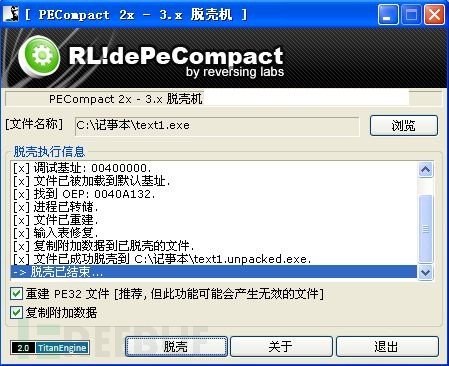将脱壳后程序再进行IDA静态分析，函数窗口可获取到所有的函数信息，主程序流程图如下所示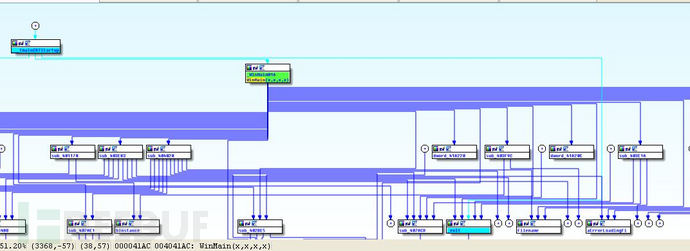### 第五步：动态调试

1、将样本1载入OD中，F9直接运行。此时，奇怪的事发生了，程序在弹出密码输入框的同时，OD左下角提示进程已经结束，这意味着程序已经运行结束，怎么密码框还在呢？！！！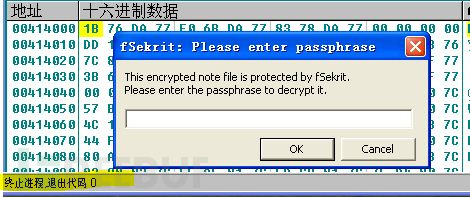2、使用文件夹的搜索功能，对全盘进行了搜索，寻找该进程对应的程序存放目录。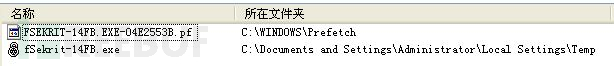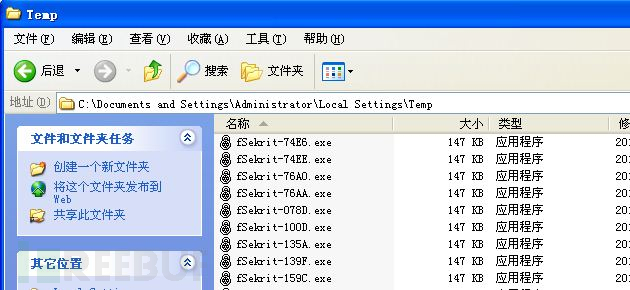3、运行这些程序，均为空白记事本，没有任何内容。经过UE比对确认，这些程序均为笔记本的原始程序，不包含任何数据。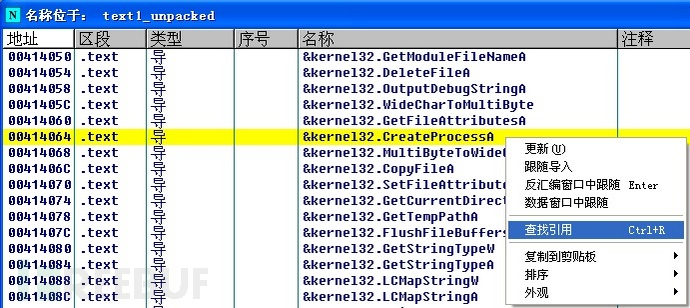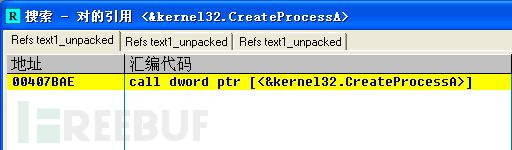407BAE处调用了该函数创建新进程，在407BAE下断点，运行程序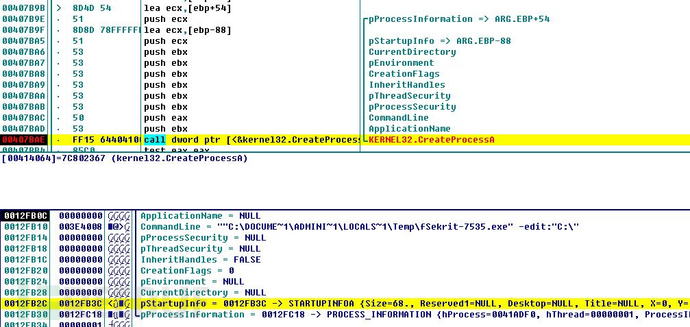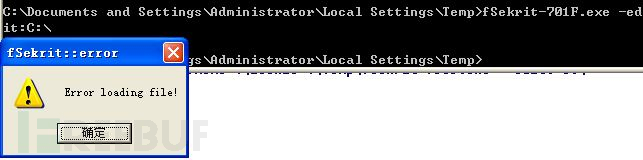## 0×04深度分析

### 1、附加程序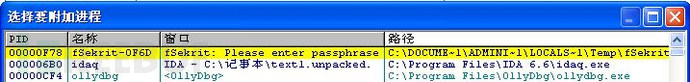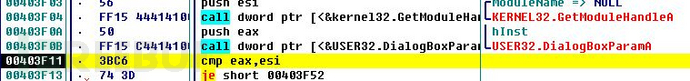### 2、IDA辅助查看程序流程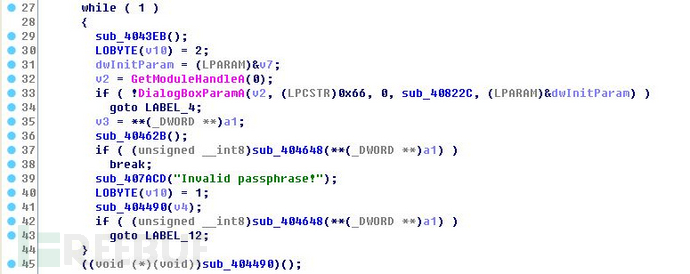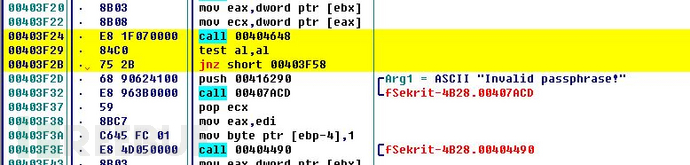repe cmps byte ptr [esi],byte ptr [edi]

ESI为12FE78，EDI为3E3D99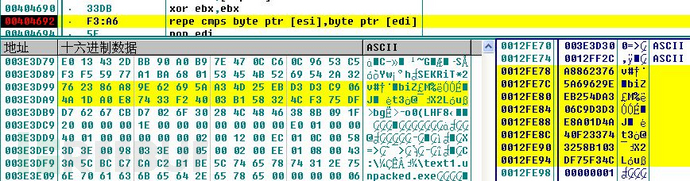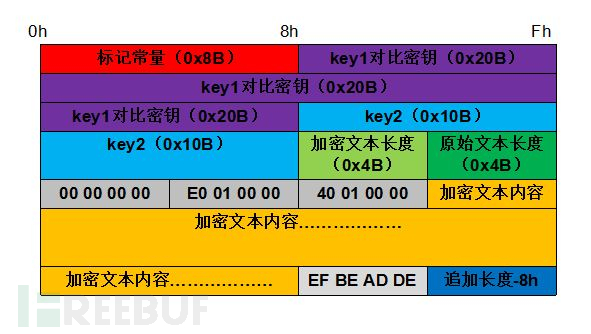### 3、加密流程

```  ```size_t __usercall sub_407481@(int a1@, void *a2, size_t a3)
{
int v3; // edi@1
size_t result; // eax@1
int v5; // ebx@1
size_t v6; // ebx@7
v3 = *(_DWORD *)a1 & 0x3F;
result = a3 + *(_DWORD *)a1;
v5 = 64 - v3;
*(_DWORD *)a1 = result;
if ( result < a3 )
++*(_DWORD *)(a1 + 4);
if ( v3 && a3 >= v5 )
{
memcpy_0((void *)(v3 + a1 + 40), a2, 64 - v3);
result = sub_404B4C(a1 + 40, a1);
a3 -= v5;
a2 = (char *)a2 + v5;
v3 = 0;
}
if ( a3 >= 0x40 )
{
v6 = a3 >> 6;
do
{
result = sub_404B4C((int)a2, a1);
a3 -= 64;
a2 = (char *)a2 + 64;
--v6;
}
while ( v6 );
}
if ( a3 )
result = (size_t)memcpy_0((void *)(v3 + a1 + 40), a2, a3);
return result;
}   ``````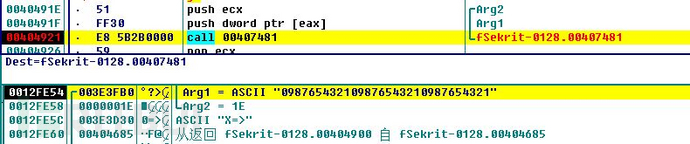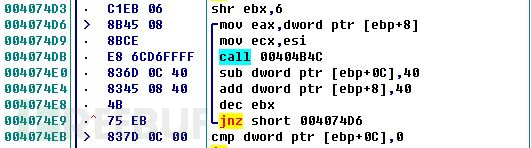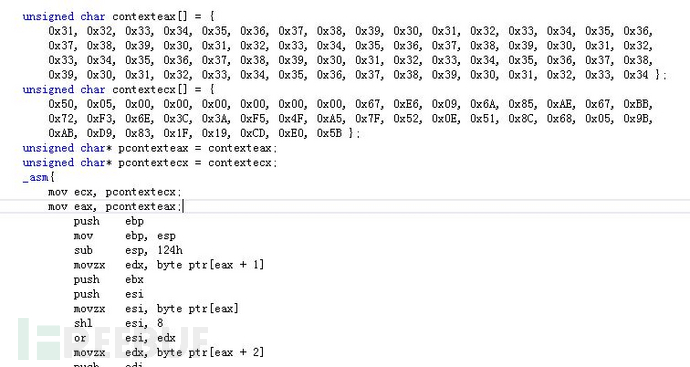404B4C函数的输入分别为eax（待加密的内容，长度为0×40字节），ecx（生成的密钥存放位置），ecx所指向的密钥存放位置为0×28字节，前8个字节存放着原始明文的总长度，后面0×20字节存储着生成密钥，且这0×20字节密钥设有初始值。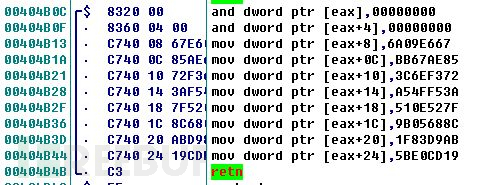407481函数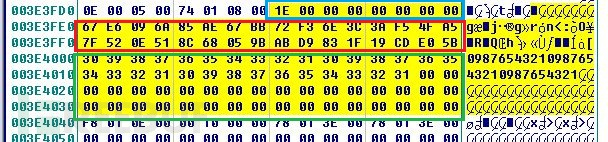407481过程表示

``` ```

`count = Arg2 / 0x40;   //明文长度除以0x40`

data=Arg1;

While(count--)

{

Call 404B4C(data); //每次讲明文的0x40字节进行加密计算

data=data+0x40;

}

Call 40B240(Arg2 %0x40 ,data);  //将明文的剩余部分写入绿色框中

上述过程结束后，程序再次调用了407481函数，参数为原始加密文件中key2密钥，长度为0×10字节。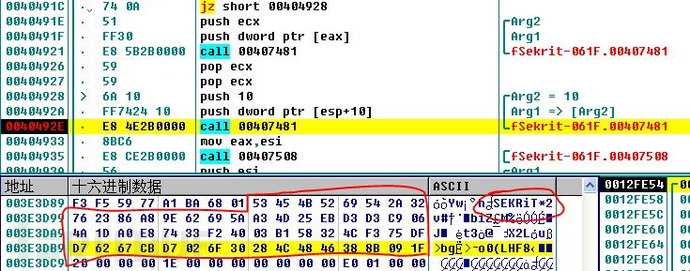407481函数运行后将0×10字节的密钥追加在了剩余明文尾部。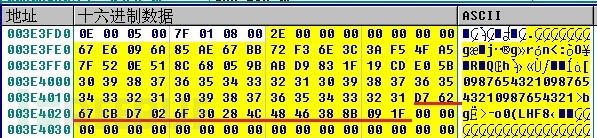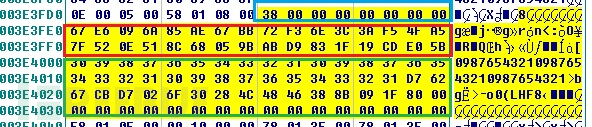1.将红色区域初始化，将初始密钥写入

2.每次读取0×40字节的原始数据，使用红色区域的密钥进行加密变换，生成的密钥输出到红色区域；

3.将剩余的原始数据进行填充处理，使其达到0×40长度，然后再进行一次密钥变换，此时生成的密钥便是比对密钥，用于和正确的密钥进行比对。

## 0×05密文解密

1、猜想：程序在获取到输入密码后，利用输入密码对密文进行解密，用解密后的密文生成对比密钥。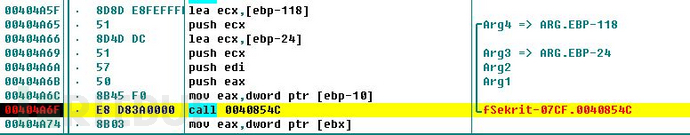40854C函数在解密过程中还调用了一些未知区域的数据进行解密变换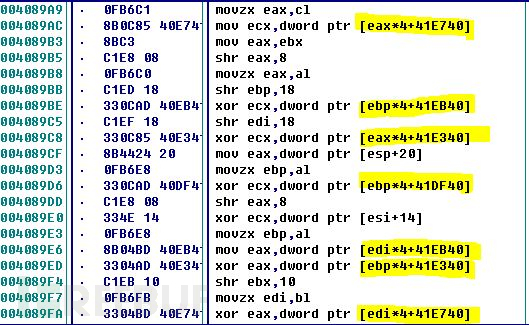### 2、猜想：程序在初始化运行时，生成了这些未知区域的数据。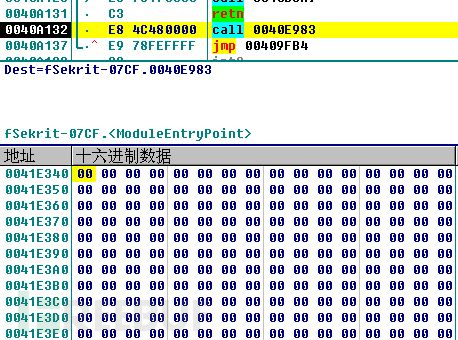在此数据区域设置内存写入断点，F9运行。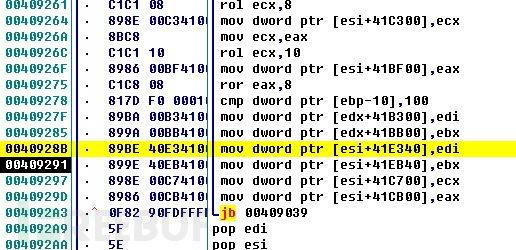```  ```int sub_408FFB()
{
int v0; // eax@1
int v1; // ecx@1
…………………………
v0 = 1;
v1 = 0;
do
{
v2 = 283 * (((unsigned int)v0 >> 7) & 1);
*(int *)((char *)&dword_41CF00 + v1) = v0;
v1 += 4;
v0 = v2 ^ 2 * v0;
}while ( (unsigned int)v1 < 0x28 );
v28 = 0;
do
{
v3 = v28;
LOBYTE(v2) = v28;
v4 = sub_408F52(v1, v2);
v5 = 2 * (v4 ^ 2 * (v4 ^ 2 * (v4 ^ 2 * v4))) ^ v4;
v6 = (unsigned __int8)(v5 ^ BYTE1(v5) ^ 0x63);
v7 = 2 * v6 ^ 283 * (v6 >> 7) | ((v6 | (v6 << 8)) << 8) | 452984832 * (v6 >> 7) ^ ((v6 ^ 2 * v6) << 24);
……………………
v20 = 72448 * v17 ^ 72448 * v18 ^ 72448 * v19 ^ ((v14 ^ 8 * v14) << 8) | 18546688 * v17 ^ 18546688 * v15 ^ 18546688 * (((unsigned int)v14 >> 6) & 1) ^ 18546688 * v18 ^ 18546688 * v19 ^ ((v14 ^ 4 * v16) << 16) | 452984832 * ((unsigned int)v14 >> 7) ^ 452984832 * v17 ^ 452984832 * v18 ^ 452984832 * v19 ^ ((v14 ^ 2 * (v14 ^ 4 * v14)) << 24) | 283 * ((unsigned int)v14 >> 7) ^ 283 * v17 ^ 283 * v15 ^ 283 * (((unsigned int)v14 >> 6) & 1) ^ 283 * v18 ^ 283 * v19 ^ 2 * (v14 ^ 2 * v16);
……………………
dword_41E340[v8] = v21;
dword_41EB40[v8] = v24;
dword_41C700[v8] = v1;
dword_41CB00[v8] = v26;
}
while ( v28 < 0x100 );
dword_41FF58 = 1;
return 0;
}   ``````

## 0×06.解密流程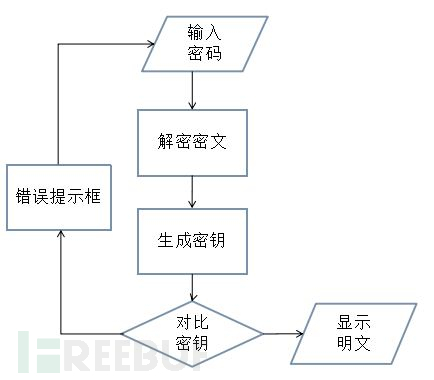## 手把手教你找回加密程序的密码

- - FreeBuf.COM | 关注黑客与极客

## 加密锁和云授权

- MArCoRQ - 月光博客
在中国，加密锁仍然是占主导地位的软件保护方式. 近30年来，计算机硬件不知更新了多少代，软件技术也从上世纪90年代起进入了互联网时代，而加密锁保护方式却一直没有改变，顶多从原先的并口锁进化到USB锁，这是为什么呢. 　　这是因为，30年来，软件的盗版依然存在，人们却没有找到应对盗版更好的办法. 另外，传统的观念认为，加密锁具有安全强度高、软件授权可随加密锁移动使用优点.

- 请叫我火矞弟 - Solidot

## 透明数据加密

- - CSDN博客数据库推荐文章

- - CSDN博客推荐文章

## 恐怖分子用单字母加密法加密通信

- Mathack - Solidot

## Android 应用程序

- - CSDN博客推荐文章
Android 应用程序由四个模块构造而成：Activity、Intent 、Content Provider 、Service. 下面简单介绍一下如下模块的含义：. 1、Activity  "活动". 一个Activity就是单独的屏幕，每一个活动都被实现为一个独立的类，并且从活动基类中继承而来，活动类将会显示由视图控件组成的用户接口并对事件作出响应.

## Linux程序调试

- - C++博客-首页原创精华区
Linux下的段错误产生的原因及调试方法    原文地址： http://www.upsdn.net/html/2006-11/775.html .    参考地址： http://www.cnblogs.com/khler/archive/2010/09/16/1828349.html .

- 可可 - 宇宙的心弦

## AES加密算法动画演示

- Charles - 酷壳 - CoolShell.cn